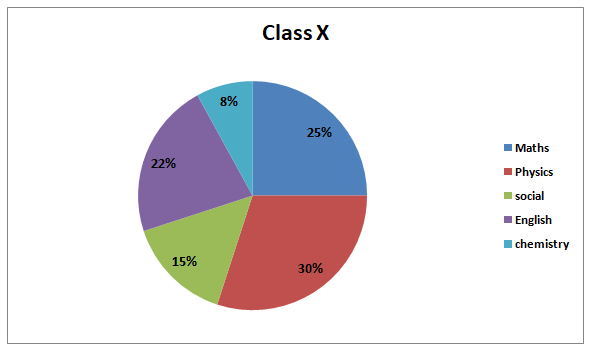# SBI Clerk Prelims 2021 Quantitative Aptitude Questions (Day-16)

Dear Aspirants, Our IBPS Guide team is providing new series of Quantitative Aptitude Questions for SBI Clerk Prelims 2020 so the aspirants can practice it on a daily basis. These questions are framed by our skilled experts after understanding your needs thoroughly. Aspirants can practice these new series questions daily to familiarize with the exact exam pattern and make your preparation effective.

Start Quiz

Pie chart

Directions (01-05): Study the following information carefully and answer the questions given below.

The Pie chart shows the percentage distribution of students like five different subjects out of total students in class X.Note: Total number of students In class X = 500

1) Find the average number of students who like Maths, social and English and physics.

A.105

B.115

C.120

D.80

E.150

2) Out of the total students who like Physics, the ratio between the boys and girls is 2:3, and then find the total number of boys who like Physics?

A.50

B.60

C.65

D.70

E.75

3) Total number of students who like social are what percentage of the total number of students who like English and chemistry together?

A.45%

B.50%

C.55%

D.39%

E.57%

4) Total number of students in class XII is 50% more than that of class X, and the ratio between boys and girls is 7:8, find the total number of boys in class XII?

A.350

B.420

C.490

D.280

E.560

5) What is the central angle of the sector corresponding to the students who like Physics?

A.180 degrees

B.108 degrees

C.63 degrees

D.54 degrees

E.118 degrees

Simplification

Directions (06-10): What value should come in the place of (?) in the following questions.

6)  (9040 ÷ 113) * 12.5 = ?

A.1000

B.1020

C.1040

D.1060

E.1200

7) (1/4) * 3040 – (2/5) * ? = 140 * 4

A.450

B.480

C.500

D.520

E.540

8) √2304 ÷ 3√(1728)  + 2√(3025)  * √16 = ?

A.224

B.228

C.232

D.236

E.240

9) 20% of 400 + ?% of 280 = 150

A.30

B.35

C.20

D.25

E.40

10) √2809 + √441 = √4096 + ?

A.8

B.10

C.12

D.15

E.18

Required average = (25 + 15 + 22 + 30)/4

= 92/4

= 23%

= 23/100 * 500

= 23 * 5

=115

Total number of students who like Physics = 30/100 * 500

= 150

Ratio between boys and girls = 2:3

Number of boys = 2/5 * 150

= 60

Number of students who like social= 15/100*500

= 75

Number of students who like English and chemistry together = 30/100*500

= 150

Hence required percentage = 75/150*100

= 50%

Number of students in class X = 500

Number of students in class XII = 150%(500)

= 150/100*500

= 750

Number of boys = 7/15*750

=350

Total 100% = 360

Physics = 30%

hence required angle = 30/100*360= 108 degrees

(9040 ÷ 113) * 12.5 = ?

80 * 12.5 = ?

? = 1000

(1/4) * 3040 – (2/5) * ? = 140 * 4

760 – 560 = ? * 2/5

? = 500

√2304 ÷ 3√(1728)  + 2√(3025)  * √16 = ?

48/12 + 55 * 4 = ?

? = 224

20% of 400 + ?% of 280 = 150

80 + ? % of 280 = 150

? = 25

√2809 + √441 = √4096 + ?

53 + 21 = 64 + ?

? = 10

 Check Here to View SBI Clerk Prelims 2021 Quantitative Aptitude Questions Day – 15 Day – 14 Day – 13